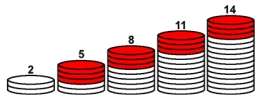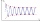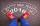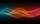# Find the sum

Find the sum of all natural numbers from 1 and 100, which are divisible by 2 or 5

s =  3050

### Step-by-step explanation:Did you find an error or inaccuracy? Feel free to write us. Thank you!## Related math problems and questions:

• Sum of the seventeen numbersThe sum of the 17 different natural numbers is 154. Determine the sum of the two largest ones.
• Consecutive numbersSum of ten consecutive numbers is 105. Determine these numbers (write first and last).
• Sum of odd numbersFind the sum of all odd integers from 13 to 781.
• The sumThe sum of the first 10 members of the arithmetic sequence is 120. What will be the sum if the difference is reduced by 2?
• 7 digit numberIf 3c54d10 is divisible by 330, what is the sum of c and d?
• Find twoFind two consecutive natural numbers whose product is 1 larger than their sum. Searched numbers expressed by a fraction whose numerator is the difference between these numbers and the denominator is their sum.
• Octahedron - sumOn each wall of a regular octahedron is written one of the numbers 1, 2, 3, 4, 5, 6, 7 and 8, wherein on different sides are different numbers. For each wall John make the sum of the numbers written of three adjacent walls. Thus got eight sums, which also
• NumbersDetermine the number of all positive integers less than 4183444 if each is divisible by 29, 7, 17. What is its sum?
• Sum of four numbersThe sum of four consecutive natural numbers is 114. Find them.
• Sum 1-6Find the sum of the geometric progression 3, 15, 75,… to six terms.
• Finite arithmetic sequenceHow many numbers should be inserted between the numbers 1 and 25 so that all numbers create a finite arithmetic sequence and that the sum of all members of this group is 117?
• CalculateCalculate the sum of all three-digit natural numbers divisible by five.
• Probably memberLook at the series 2,6,25,96,285, ? What number should come next?
• Three intsThe sum of three consecutive integers is 2016. What numbers are they?
• The sumThe sum of the squares of two immediately following natural numbers is 1201. Find these numbers.
• DivisibilityWrite all the integers x divisible by seven and eight simultaneously, for which the following applies: 100 < x < 200.
• Three numbersThe sum of the three numbers is 287. These numbers are in a ratio of 3: 7: 1/4. Find these numbers.